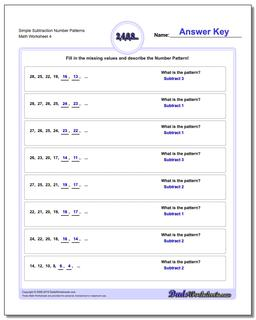# Math Worksheets: Number Patterns: Number Patterns: Simple Subtraction Number Patterns (Fourth Worksheet)## Simple Subtraction Number Patterns (Fourth Worksheet)

PropertyValue
DescriptionSimple Subtraction Number Patterns: Number pattern problems using only subtraction operations. (Fourth Worksheet)
Resource TypeWorksheet